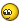Полная версия: Определить принадлежит ли точка (x,y) области
Rikki_Irena
Составить программу , используя условный операторНажмите для просмотра прикрепленного файла
Krjuger
Покажите что не получаеться, в чем проблема и что подразумевается под закрашенными облостями? Вывести множество значений? Вывести те точки которые попадают в данные области?Более точно условие опишите
Rikki_Irena
вот первую решила с уравнением, а вторую не знаю даже как
Krjuger
Ну вот это уже что-то)))
`Varx,y: integer;beginx:=3;y:=6;if ((x-5>=0) and (y<=x) and (y-5>=0)) or ((x-5<=0) and (y>=x) and (y-5<=0)) then  writeln('DA')elsewriteln('NET');readln;end.`

Вроде работает))))

МОжно раскидать по нескольким условиям,если есть вопросы,спрашивайте...
Rikki_Irena
Алексей, спасибо большое !!!!^^order prednisone online from ind
Can You Take Amoxicillin With Lyica
can you buy zithromax over the c
La Nueva Viagra